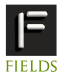11-15 July 2011 INTERNATIONAL CONFERENCE ON SCIENTIFIC COMPUTATION AND DIFFERENTIAL EQUATIONS SciCADE 2011 hosted by the Fields Institute, held at the University of TorontoProgram New Talent Award Toronto Information Contact Us gensci(PUT_AT_SIGN_HERE)fields.utoronto.caMINISYMPOSIA Numerical PDE Methods for Mathematical Finance Organized by Ken Jackson, Computer Science Department, University of Toronto

Many problems in mathematical finance can be modeled by PDEs, most of which cannot be solved in closed form. Therefore, numerical methods are needed to solve them. As mathematical finance has developed over the past 30 or so years, the PDE models have become more sophisticated and the associated numerical problems more challenging. This minisymposium consists of a sample of four talks in this area.

 SPEAKERS Peter Forsyth Cheriton School of Computer Science, University of Waterloo Methods for Pricing American Options Under Regime Switching We analyze a number of techniques for pricing American options under a regime switching stochastic process. The techniques analyzed include both explicit and implicit discretizations with the focus being on methods which are unconditionally stable. In the case of implicit methods we also compare a number of iterative procedures for solving the associated nonlinear algebraic equations. Numerical tests indicate that a fixed point policy iteration, coupled with a direct control formulation, is a reliable general purpose method. Finally we remark that we formulate the American problem as an abstract optimal control problem, hence our results are applicable to more general problems as well. Stephen Tse Cheriton School of Computer Science, University of Waterloo Comparison between the Mean Variance optimal and the Mean Quadratic Variation optimal trading strategies We compare optimal liquidation policies in continuous time in the presence of trading impact by numerical solutions of Hamilton Jacobi Bellman (HJB) partial differential equations (PDE). We show quantitatively that the mean-quadratic-variation strategy can be significantly suboptimal in terms of mean-variance efficiency and that the mean-variance strategy can be significantly suboptimal in terms of mean-quadratic-variation efficiency. Moreover, the mean-quadratic-variation strategy is on average more suboptimal than the mean-variance strategy, in the above sense. In the semi-Lagrangian discretization used for solving the HJB PDEs, we show that interpolating along the semi-Lagrangian characteristics results in significant improvement in accuracy over standard interpolation while still guaranteeing convergence to the viscosity solution. Christina Christara Computer Science Department, University of Toronto PDE modeling and pricing of Power Reverse Dual Currency swaps Cross-currency interest rate derivatives in general, and foreign exchange (FX) interest rate hybrids in particular, are of great practical importance. In particular, Power Reverse Dual Currency (PRDC) swaps are one of the most widely traded and liquid FX interest rate hybrids. These products are exposed to moves in both the spot FX rate and the interest rates of the domestic and foreign currencies. We discuss the modeling of PRDCs using two one-factor Gaussian models for the two stochastic interest short rates, and a one-factor FX skew model with a local volatility function for the spot FX rate. The resulting time-dependent PDE is three-dimensional in space, a fact that makes the pricing computationally challenging. We study two numerical techniques for solving the PDE. The first uses Alternating Direction Implicit (ADI) timestepping and the second a standard Crank-Nicolson (CN) discretization in time, while both use centered differences in space. The ADI technique requires solutions of tridiagonal systems, while the CN discretization requires solution of block-banded systems. The latter are treated by GMRES with FFT-based preconditioning. Numerical results indicate that the ADI method is faster in absolute terms, while both methods are optimal or almost optimal in asymptotic complexity. Duy Minh Dang Computer Science Department, University of Toronto Pricing long-dated foreign exchange interest rate hybrids via a PDE approach with an efficient implementation on Graphics Processing Units Power Reverse Dual Currency (PRDC) swaps are popular long-dated foreign exchange (FX) interest rate hybrids. A vanilla Power Reverse Dual Currency (PRDC) swap, similar to a vanilla fixed-for-floating single-currency swap, involves an agreement between the issuer and the investor, in which the issuer pays the investor a stream of PRDC coupon amounts, and, in return, receives the investor's domestic LIBOR payments. However, the PRDC coupons are linked to the spot FX rate prevailing when the PRDC coupon rate is set. Variations of PRDC swaps with exotic features, such as Bermudan cancelable, or knockout, or FX Target Redemption (FX-TARN), are much more popular than ``vanilla'' PRDC swaps. The modeling of PRDC swaps using one-factor Gaussian models for the domestic and foreign interest short rates, and a one-factor skew model for the spot FX rate results in a time-dependent parabolic PDE in three space dimensions. While solution of the PDE arising from vanilla PRDC swaps presents by itself a computational challenge, the exotic features significantly increase the complexity of the pricing. In this talk, we discuss the pricing via a PDE approach of PRDC swaps with popular exotic features, namely Bermudan cancelable and FX-TARN. Our PDE pricing framework is based on partitioning the pricing problem into several independent pricing subproblems over each period of the swap's tenor structure, each of which requires a solution of the model-dependent PDE. Finite differences and the Alternating Direction Implicit (ADI) method are used for the spatial and time discretizations, respectively, of this PDE. To handle the increased computational requirements due to the exotic features, we develop a Graphics Processing Unit (GPU) parallelization of the pricing procedure on a multi-GPU platform. Numerical results demonstrating the efficiency and accuracy of the parallel numerical methods are provided.